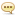Tutorials :

# Udemy - Zero To Hero! Learn Algebra Groupings And Like Terms

Author: Baturi   |   04 December 2021   |: 0MP4 | Video: h264, 1280x720 | Audio: AAC, 44.1 KHz, 2 Ch
Genre: eLearning | Language: English | Duration: 20 lectures (2 hour, 47 mins) | Size: 725 MB
Learn & practice Algebra today; groupings, like / unlike terms, brackets, polynomials, base, coefficients, powers

What you'll learn
In algebra terms are separated by a plus (+) and minus (-) only
Like terms refer to terms that have the same variables (or none) and the variables all have the same degree (power) when compared between the terms
Grouping is the process of organising and then adding/subtracting like terms
Like Terms can be added together or subtracted from each other, but we cannot perform these operations with unlike terms
Grouping like terms is the process of ordering them together and then adding/subtracting them
Circular brackets means value are excluded from a range
Square brackets means values are included in a range
Curly brackets can be used in set notation to represent a range of values
In expressions and equations the three brackets are used to separate terms or 'parts' with all given the same level of priority
Use different brackets to make the expression or equation look neater and easier to read. The type of bracket does not indicate the order in which we solve
The Distributive Law (and possibly Exponential Laws) are used to 'remove' the innermost brackets
A polynomial is created through the addition and subtraction of terms
The degree of a polynomial is equal to the highest value of the sum of the exponents of the variables in any induvial term of the polynomial
A polynomial can be characterised by the number of terms it contains:
An expression has to follow a set of strict rules in order for it to be called a polynomial
Requirements
You will need to understand basic maths concepts like division, multiplication, subtraction and addition
Description
In this course you will get professional maths tutoring to help you be successful at Algebra. We cover the basics of algebra such as like terms and grouping, as well as brackets, polynomials, base, coefficients, powers. You will get practical, step by step explanations, practice examples and demonstration lessons. A solid introduction to algebra is so important for the rest of your maths journey so join us in this course as we help you achieve your goals.
This course is engaging, practical and takes you on a powerful journey that supports your journey as a student.
Get the following when you enrol:
Engaging lessons that teach you key concepts and build up your confidence so you can apply what you've learnt
Practice questions for each concept so you can take action and apply what you have learnt and make sufficient progress
Downloadable worksheets that you can refer to and get the answers and explanations you need for future reference
Access to Kyle De Vos at any time through the messaging and assignments feature of the platform
Encouragement from other students who are focused on the same goal, just like you
Enrol today and we look forward to seeing your success as a maths student!
Who this course is for:
This course is for high school maths students
Anyone who needs a refresher on algebra basics or an introduction to algebra